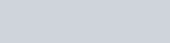The conjugate base of hydrazoic acid is :-
Question:

The conjugate base of hydrazoic acid is :-

1. $\mathrm{HN}_{3}^{-}$

2. $\mathrm{N}_{3}^{-}$

3. $\mathrm{N}_{2}^{-}$

4. $\mathrm{N}^{-3}$

Correct Option: , 2

Solution: